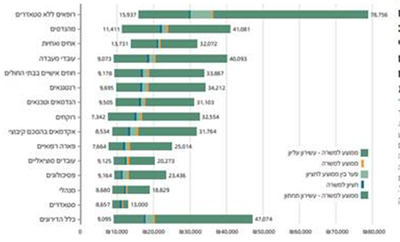## another help in graphs

hi,

this is the graph I need to build1. how do I add several lines on the bar?

2. how do I add min and max values as the side of the bar?

1 ACCEPTED SOLUTION

Accepted Solutions

## Re: another help in graphs

Use a HIGHLOW plot and stack by the grouping variable. This is sometimes called a "swimmer plot." Here are some fake data and an example:

``````/* simulate some data */
data Have;
call streaminit(1);
do Subject = 1 to 5;
startTime=rand("Poisson", 10);
do stage = 'A','B','C';
length = rand("Poisson", 7);
EndTime = StartTime + length;
output;
startTime = EndTime;
end;
end;
run;

/* create a swimmer plot for each subject; stack by stages */
proc sgplot data=Have;
highlow y=Subject low=StartTime high=EndTime / type=bar group=stage fill;
run;
``````
3 REPLIES 3

## Re: another help in graphs

Spoiler

@IditMaor wrote:

1. how do I add several lines on the bar?

2. how do I add min and max values as the side of the bar?

What kind of several lines? Where? Where would the values of the "lines" come from.

I am not sure what you mean by "min and max values as the side of the bar" at all.

Example of the data you have as a starting point is a good idea. Sometimes the way your current data is structured may not be the form needed for making nice graphs. So the first step might be to reshape your data.

Instructions here: https://communities.sas.com/t5/SAS-Communities-Library/How-to-create-a-data-step-version-of-your-dat... will show how to turn an existing SAS data set into data step code that can be pasted into a forum code box using the </> icon or attached as text to show exactly what you have and that we can test code against.

## Re: another help in graphs

Use a HIGHLOW plot and stack by the grouping variable. This is sometimes called a "swimmer plot." Here are some fake data and an example:

``````/* simulate some data */
data Have;
call streaminit(1);
do Subject = 1 to 5;
startTime=rand("Poisson", 10);
do stage = 'A','B','C';
length = rand("Poisson", 7);
EndTime = StartTime + length;
output;
startTime = EndTime;
end;
end;
run;

/* create a swimmer plot for each subject; stack by stages */
proc sgplot data=Have;
highlow y=Subject low=StartTime high=EndTime / type=bar group=stage fill;
run;
``````